## The Data: an Overview

data = readRDS("data/prepd.v3.rds")
symptoms = c("Acne", "Asthma", "ADHD", "Burn", "Erectile_dysfunction", "Scar",
"Alcoholism", "Anxiety", "Depression", "Dermatitis",
"Sleep_disorder", "Obesity")

The data contain 100 weeks worth of data, collected between 2020-03-02 and 2022-01-24, on 15 different medical conditions. The data are organised in a multivariate time serie for each of 2879 counties spread over 50 US states.

All time series are observed over the whole time period.

In what follows we focus on the 12 medical conditions above, spanning dermatological and psychiatric conditions.

data = data[data$county %in% names(which(table(data$county) > 35)), ]
data$county = droplevels(data$county)
data = data[, c("state", "county", "date", symptoms)]

The data originally contained missing data, either single data points or whole time series for specific counties and periods of time. Hence we explore the imputation error of three approaches to impute missing values in time series: moving average (with a window of 10 data points), interpolation and Kalman filters. We estimate the relative imputation error by removing individual values from the data, reimputing them and measuring the relative difference.

experiment = function(each.county, data, m, imputeFUN, symptoms) {

complete = incomplete = as.matrix(data[data$county == each.county, symptoms]) how.many = ceiling(m * length(complete)) incomplete[sample(length(complete), how.many)] = NA # for each symptom individually. for (s in colnames(incomplete)) { # impute using the user-provided function FUN; short time series cannot # be imputed, just return zero average error. imputed = try(imputeFUN(incomplete[, s]), silent = TRUE) if (is(imputed, "try-error")) return(0) else incomplete[, s] = imputed }#FOR reldiff = abs(complete - incomplete) / complete reldiff[is.na(reldiff) | is.infinite(reldiff)] = 0 return(sum(reldiff) / how.many) }#EXPERIMENT All the considered methods perform similarly for all of 2% and 5%, 10% and 20% proportions of missing values.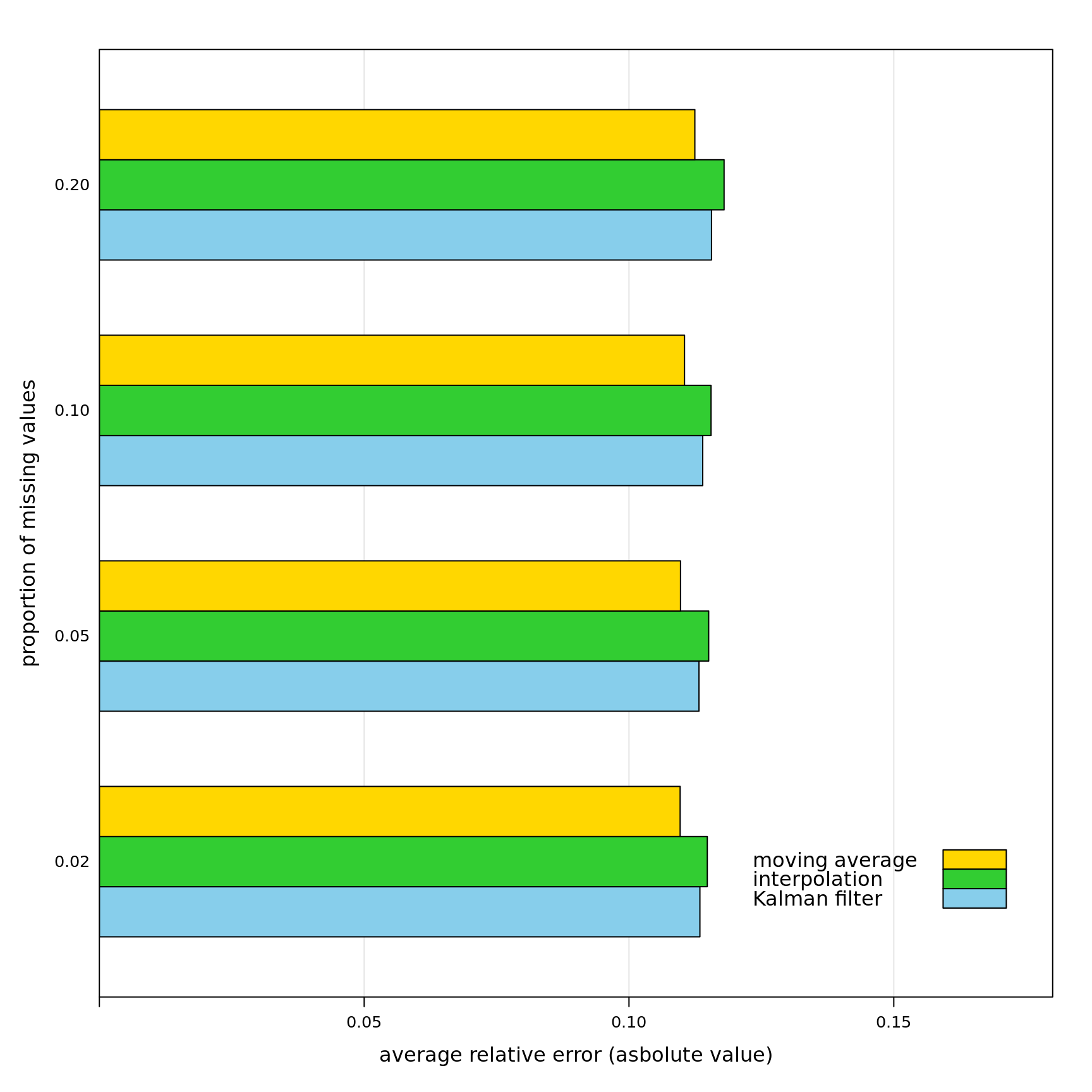We perform a similar experiment for values missing in a block of consecutive data points, like symptoms not being recorded for a whole month in a specific county. experiment = function(each.county, data, m, imputeFUN, symptoms) { complete = incomplete = as.matrix(data[data$county == each.county, symptoms])

which.symptoms = sample(ncol(complete), ceiling(m * length(complete)), replace = TRUE)
which.times = sample(nrow(complete) - 3, ceiling(m * length(complete)), replace = TRUE)
for (s in which.symptoms)
incomplete[which.times[s] + 0:3, s] = NA
how.many = sum(is.na(incomplete))

# for each symptom individually.
for (s in colnames(incomplete)) {

# impute using the user-provided function FUN; short time series cannot
# be imputed, just return zero average error.
imputed = try(imputeFUN(incomplete[, s]))
if (is(imputed, "try-error"))
return(0)
else
incomplete[, s] = imputed

}#FOR

reldiff = abs(complete - incomplete) / complete
reldiff[is.na(reldiff) | is.infinite(reldiff)] = 0

return(sum(reldiff) / how.many)

}#EXPERIMENT

All the considered methods perform similarly for all of the considered proportions of missing values.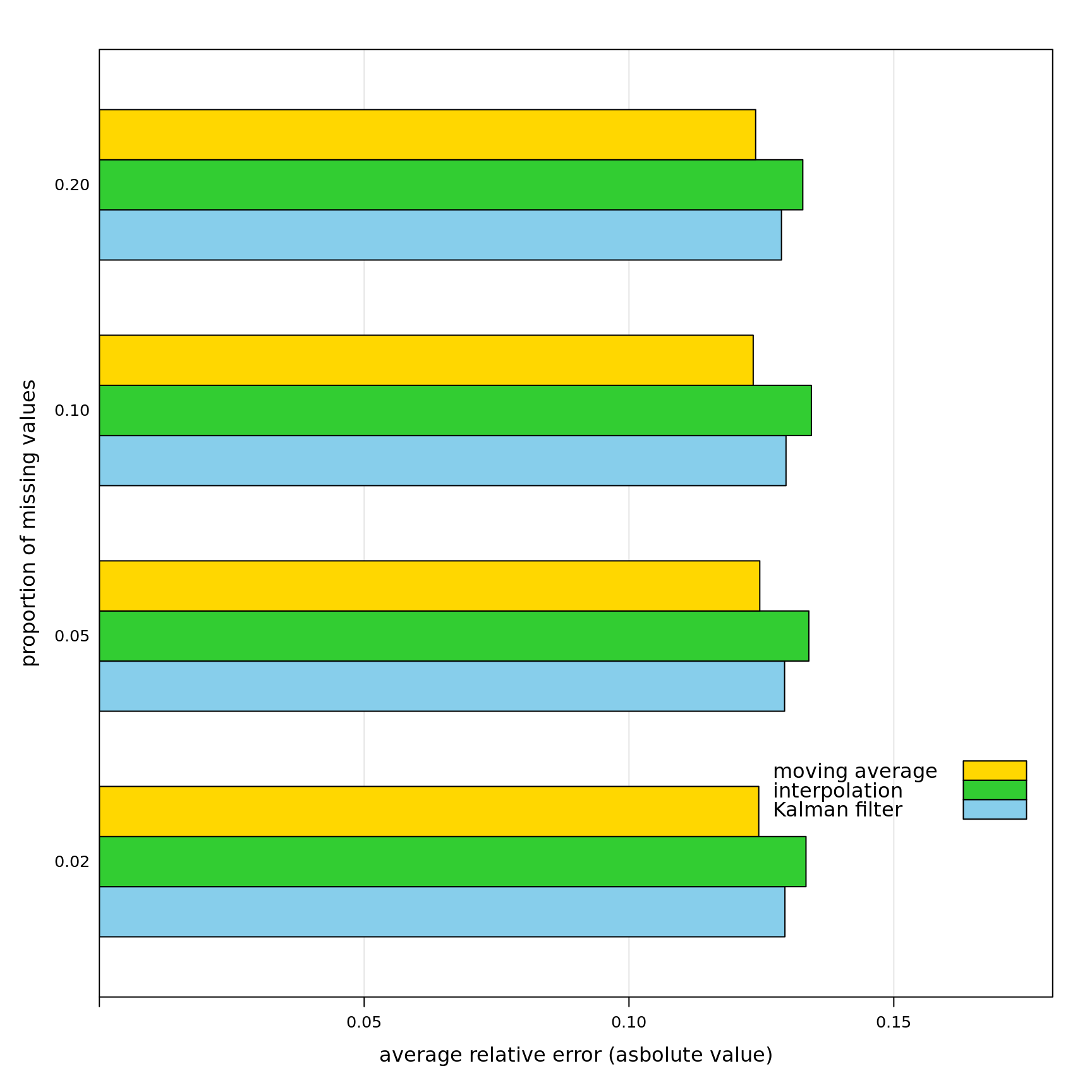## Dependence Structure of the Data

The data are characterised by a strong dependence between observations across both space and time. In the case of space, we can measure how much of the variance of the conditions of interest is explained by the US states and the counties:

r2.states = parSapply(cl, symptoms, function(symptom, data) {

model = lmer(paste(symptom, "~ 1 | state"), data = data)
return(1 - var(resid(model)) / var(data[, symptom]))

}, data = data[, c("state", "date", symptoms)])

r2.counties = parSapply(cl, symptoms, function(symptom, data) {

model = lmer(paste(symptom, "~ 1 | county"), data = data)
return(1 - var(resid(model)) / var(data[, symptom]))

}, data = data[, c("county", "date", symptoms)])

We compute the proportion of explained variance using an intercept-only random-effect model because the heterogeneity between states and between counties violates the homoschedasticity assumption of fixed-effect models. We can see that US states explain between 8% and 16% of the variance in each of the symptoms (average 12%); counties explain between 26% and 84% (average 51%).

To account for time dependence, we can add an autocorrelation with lag 1 to the individual time series corresponding to the different symptoms in the random-effect model for the counties. The implied assumption is that each symptom depends on the itself one week prior within each county.

r2.time = parSapply(cl, symptoms, function(symptom, data) {

model = lmer(paste(symptom, "~ 1 | county"), data = data)
rr = residuals(model)
date = as.integer(as.factor(data\$date))
model = try(lme(rr ~ 1, random = ~ 1 | date, correlation = corAR1(),
control = lmeControl(returnObject = TRUE)))

return(1 - var(resid(model)) / var(data[, symptom]))

}, data = data[, c("county", "date", symptoms)])

Time and space dependence together explain between 35% and 86% of the variance in each of the symptoms (average 63%). This indicates that a non-negligible part of the information in the symptoms is a result of the data points being collected as longitudinal measures in each US state.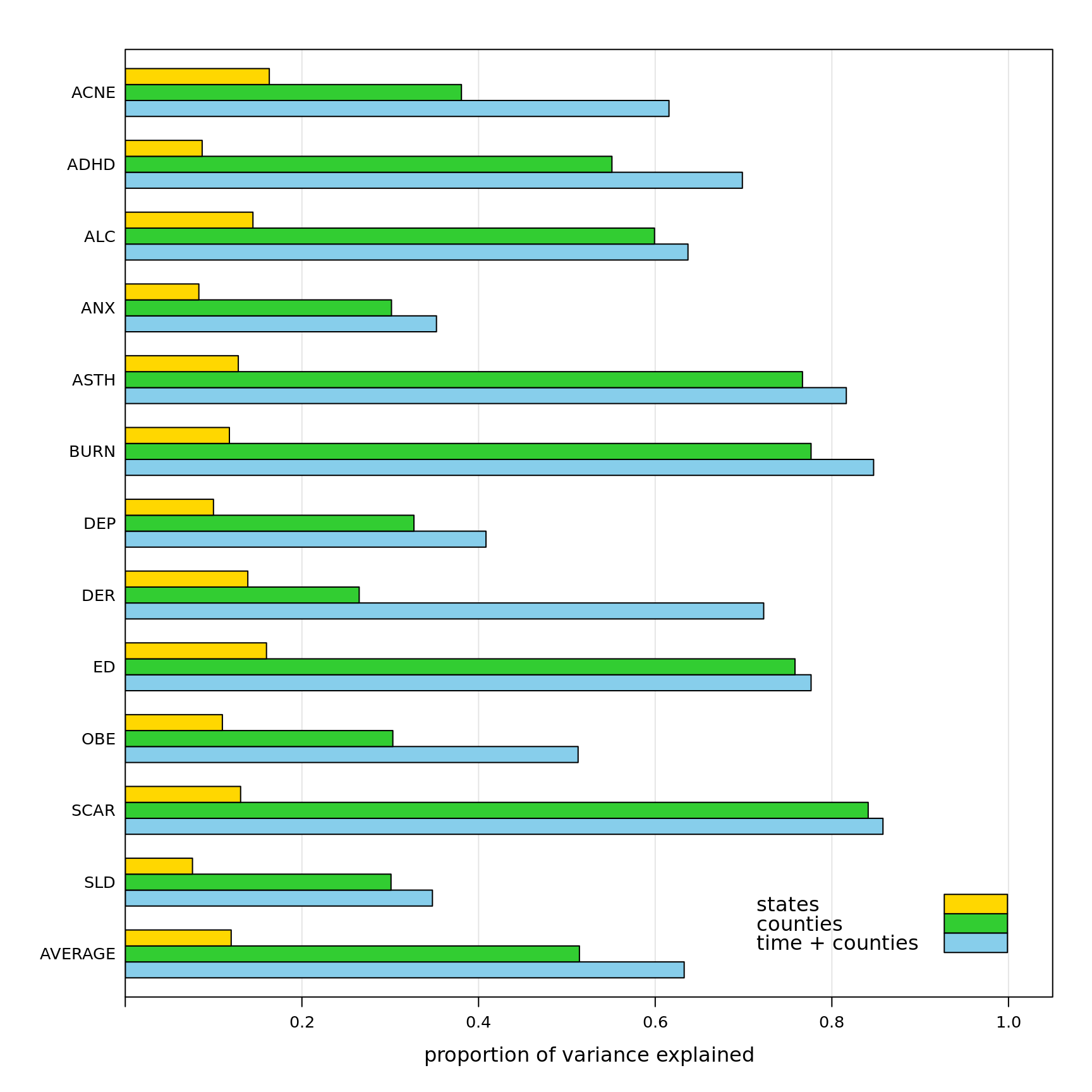## Bayesian Networks: Static versus Dynamic Structures

The classic (static) formulation of Bayesian networks is as follows: for a set of variables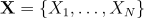and a directed acyclic graph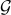, the joint distribution of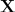can be written as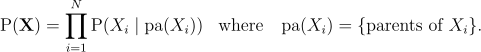Each node in the graphcorresponds to one of the variables in, and the arcs indetermines which other variables each variable depends on. If all the variables are continuous, as is the case for the medical conditions in the data, we typically assume thatfollows a multivariate normal distribution. As a result, the local distributions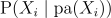take the form of a linear regression model in which the node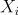is the response variable and its parents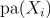are the explanatory variables: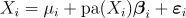where the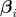are the regression coefficients associated with the parentsand the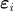are independent, normally distributed errors with some variance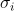specific to. If a node has not parents, the equation above will contain only the intercept and the error terms.

The assumption that residuals are independent, which implies that data points are independent, is violated by the data: as we noted above, the data points are dependent across time and space due to how they were collected. Replacing linear regression models with more complex regressions such as the random-effect models we used above makes learning the graphfrom data computationally unfeasible for large data sets. However, we can use the dynamic formulation of Bayesian network. It is constructed as follows:

1. Include two nodes for each variablein the graph: one for the variable as observed at a time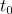and one for the variable as observed at the following time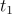.
2. Only allow arcs going from nodes at timeto nodes at timein the graph. Arcs between nodes associated with the same time point, sometimes called “instantaneous dependencies”, describe probabilistic dependencies that take effect without any time lag. Such dependencies are difficult to interpret as causal effects because Granger causality requires a time lag between cause and effect.

Arcs between nodes corresponding to the same variable at different time points model autocorrelation with lag 1: an arc from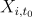to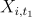implies thatis regressed against itself at the previous time point like in an AR(1) time series. Crossing arcs model feedback loops: if we both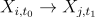and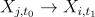are in the graph then the relationship between the underlying variables is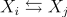. Note that static Bayesian networks are unable to express feedback loops because nodes are not duplicated and the graph is acyclic by definition.

The local distributions